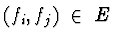CSE 5311 Fall 1999

Quiz #8

1.
(8 points.) You are given experimental data consisting of small DNA fragments. For each fragment f, it has been determined experimentally that certain fragments lie to the left of f on the chromosome, certain fragments lie to the right of f, and the rest can be on either side. How would you find a consistent ordering of the fragments from left to right that satisfies all the constaints?

Define a directed graph G=(V,E) where V represents the set of DNA fragments. Let fi, fj be in V. Edgeiff fragment fi lies to the left of fj. A consistent ordering can be found by a topological sort.

2.
(12 points.) Suppose graph G has two edge-disjoint spanning trees (two spanning trees that have no edges in common). Prove that in this graph G, every pair of nodes forms part of a cycle.

Recall that a spanning tree is a subset of edges that forms a tree connecting all nodes of the graph. If there are two edge-disjoint spanning trees, color the edges of one tree red and the edges of the other blue. For any two nodes, u and v, there is a red path between them in the first tree. However, there is also a blue path between u and v. Because there are two distinct paths (sharing no edges) between u and v, the nodes are on a cycle in graph G.